# Blackboard Shots with Prefix "AKT17"

AKT17 is 2017 MAT 1350 - Algebraic Knot Theory.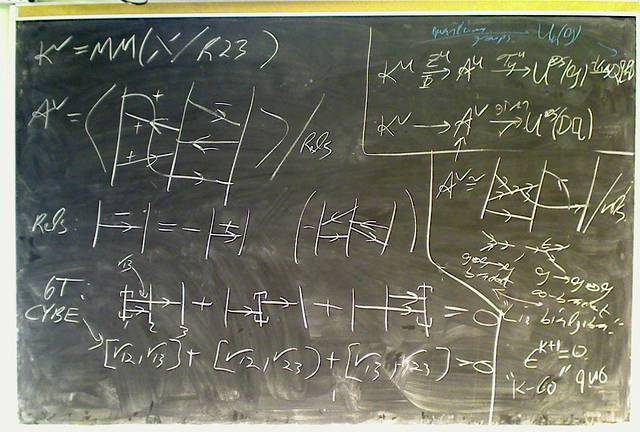170404-151431: Virtual knots (3).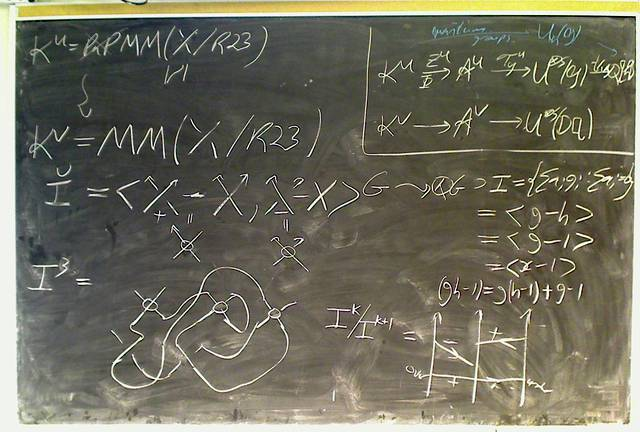170404-145038: Virtual knots (2).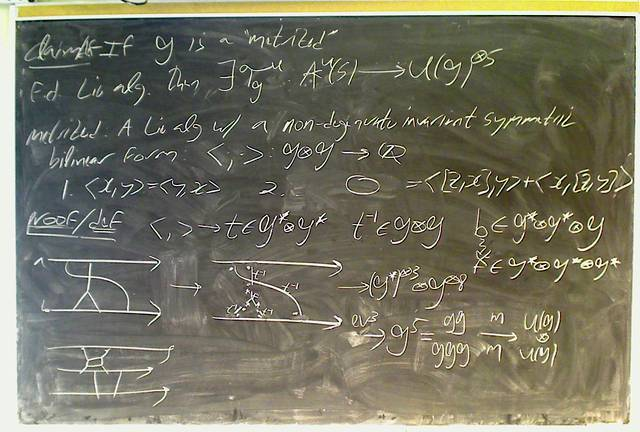170404-140623: The tensor construction (2).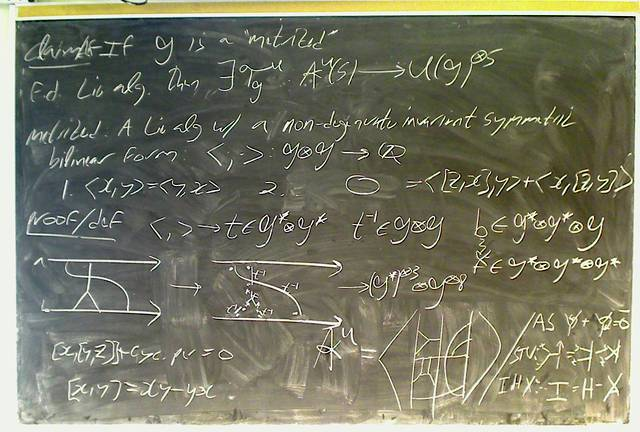170404-135940: The tensor construction.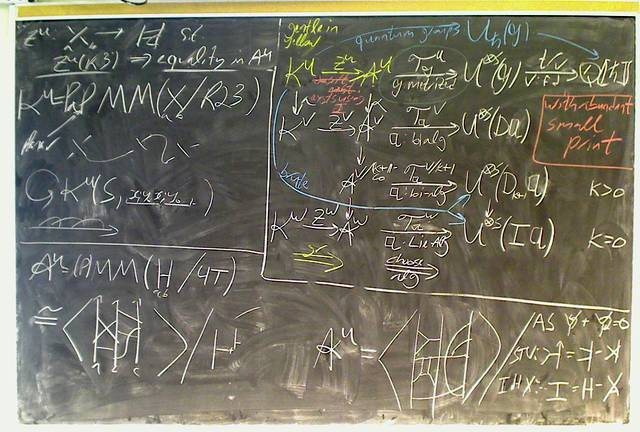170404-134421: A master diagram (2).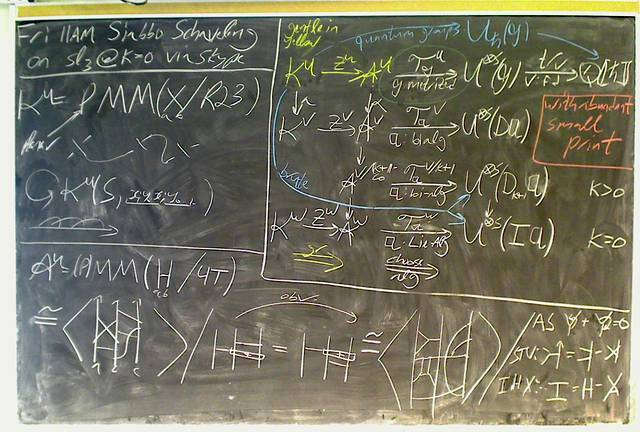170404-133657: A master diagram (1).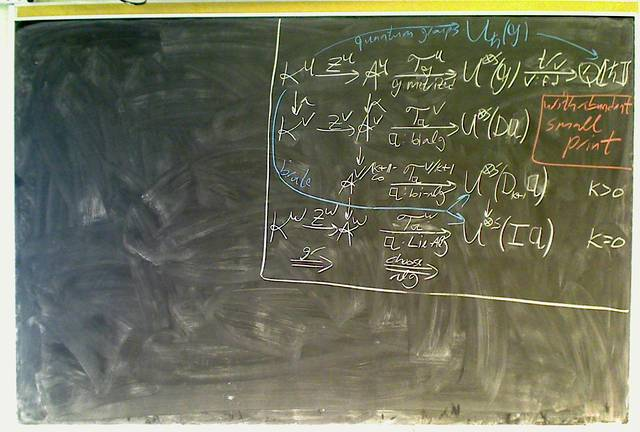170404-112811: A master diagram (0).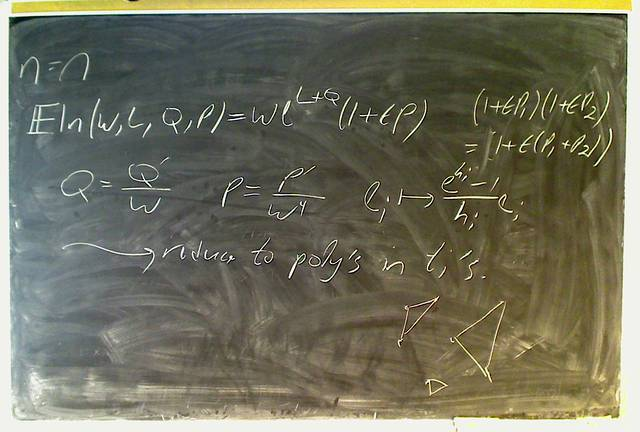170321-140437: Ugly changes of variables.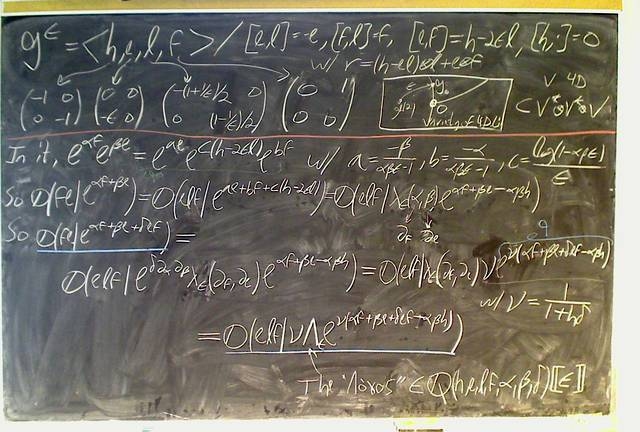170317-120957: Deriving the Logos. (more...)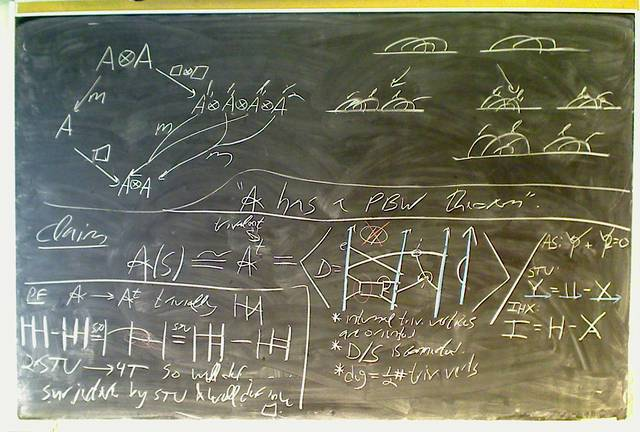170310-120220: Trivalent diagrams.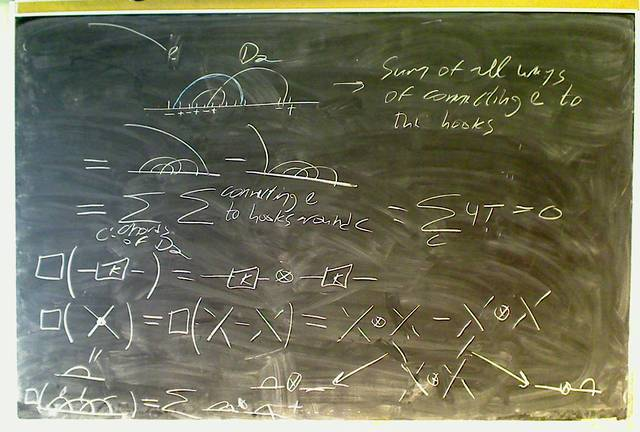170310-113620: Algebraic Structure on ${\mathcal A}$ (2).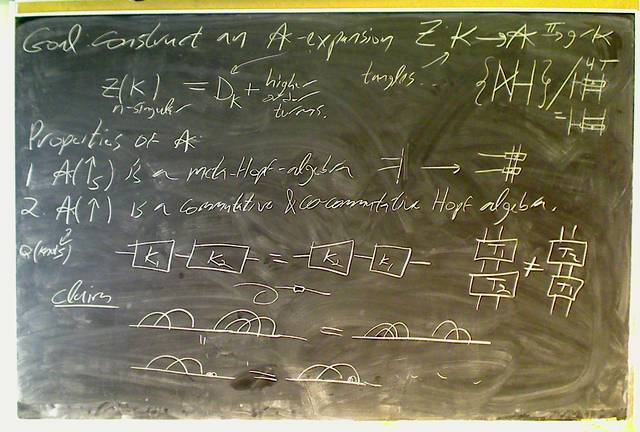170310-112539: Algebraic Structure on ${\mathcal A}$.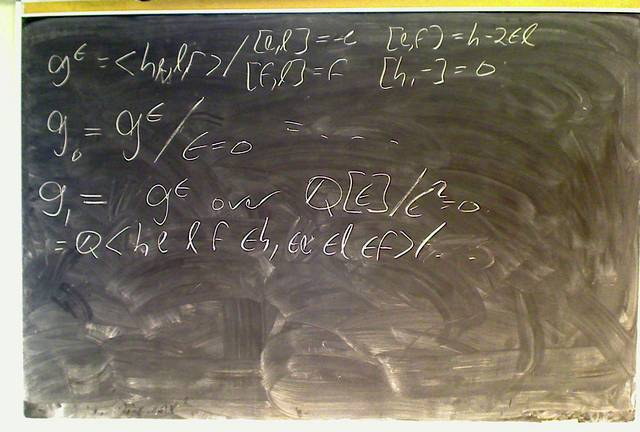170307-140325: ${\mathfrak g}_1$.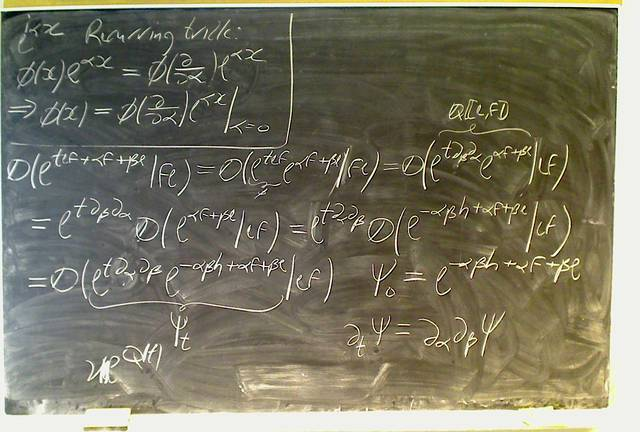170303-120237: The main ${\mathfrak g}_0$ theorem and lemmas (2).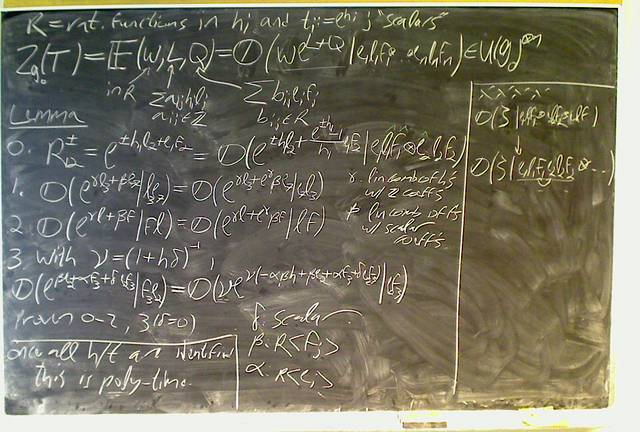170303-114018: The main ${\mathfrak g}_0$ theorem and lemmas.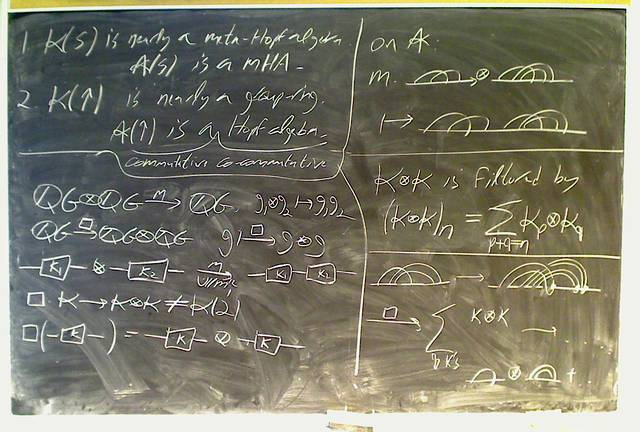170228-150154: The Hopf algebra structure on ${\mathcal A}^u(\uparrow)$.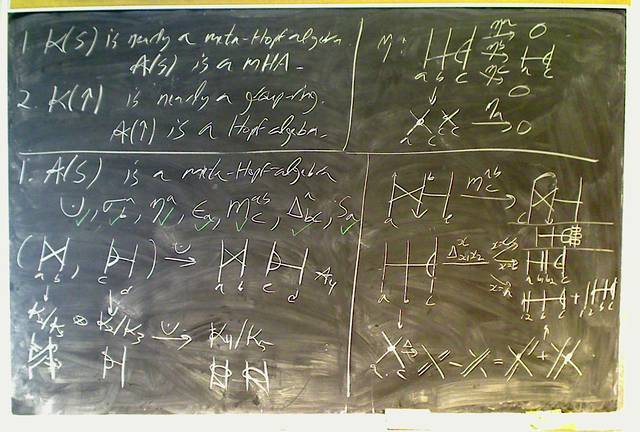170228-144905: The meta-Hopf-algebra structure on ${\mathcal A}^u(S)$.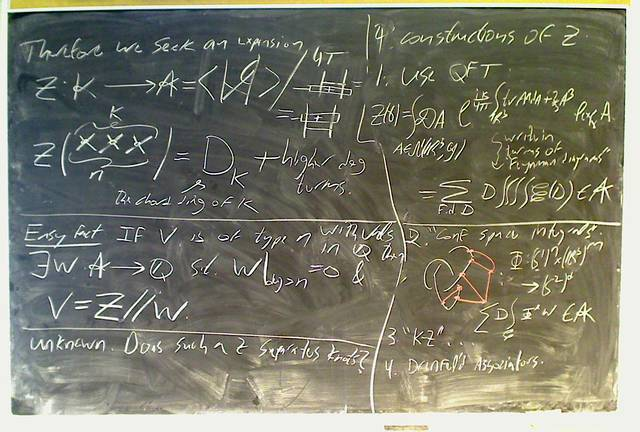170228-141323: Proofs of the fundamental theorem.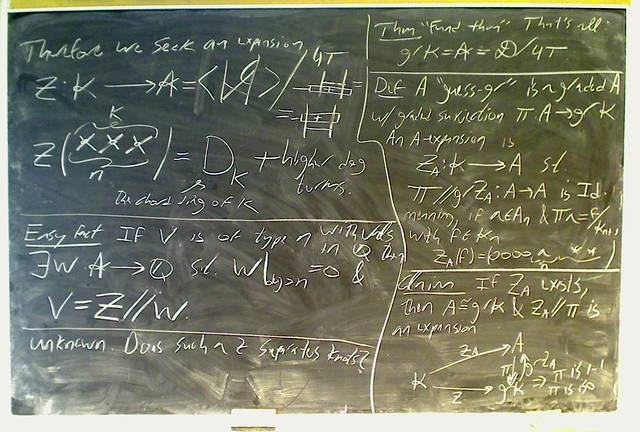170228-140501: More on A-expansions, $Z$ in the case of tangles.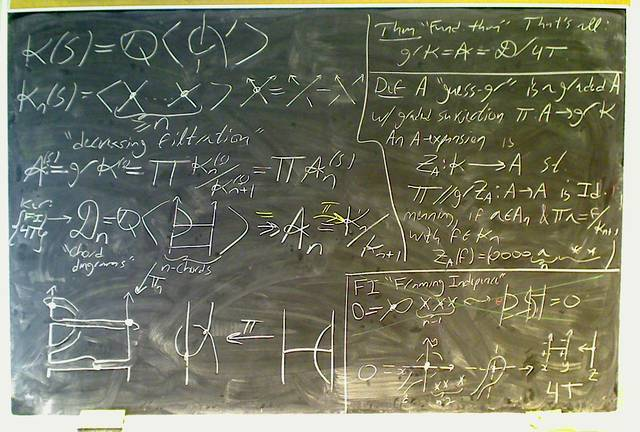170228-135002: Review, FI, 4T, A-expansions.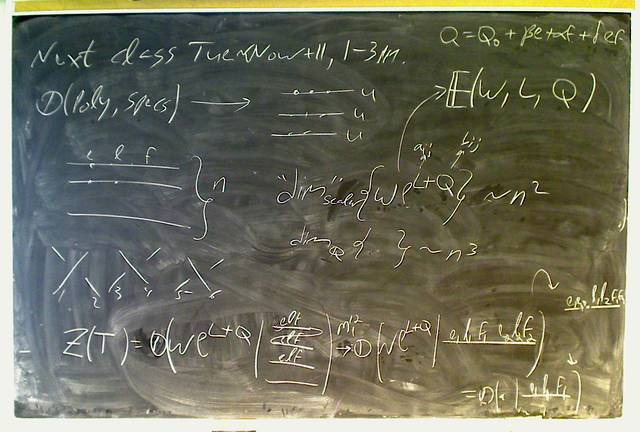170217-121428: Normal orderings and the ${\mathfrak g}_0$ invariant.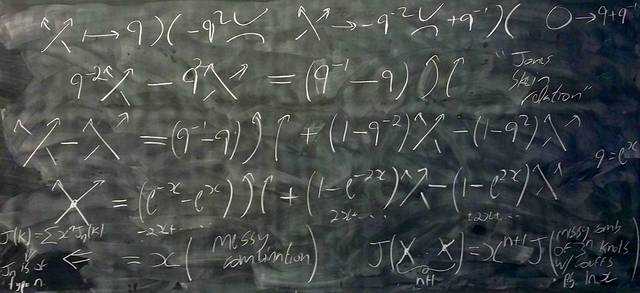170214-130108: Jones is a power series of finite type invariants.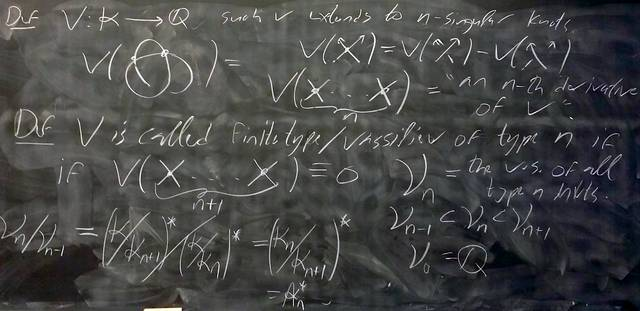170214-123504: Finite type invariants.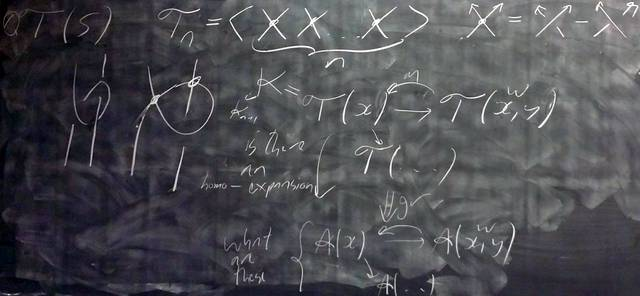170214-123457: Expansions for tangles.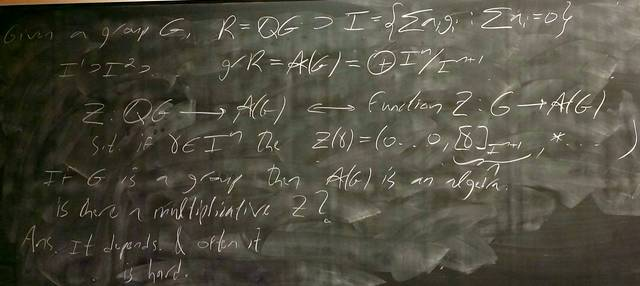170214-120427: Expansions for groups.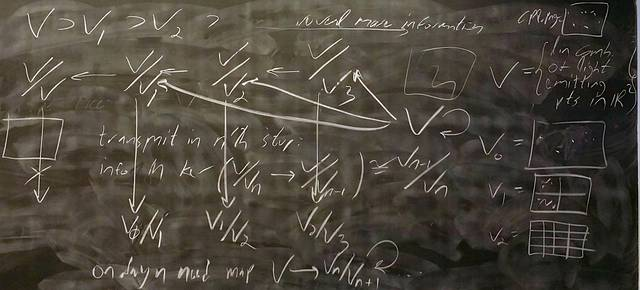170214-115607: Progressive scanning.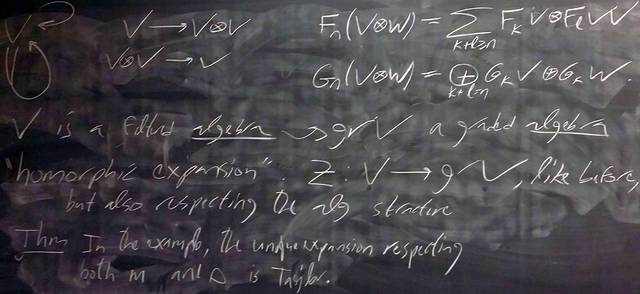170214-115601: Homomorphic expansions.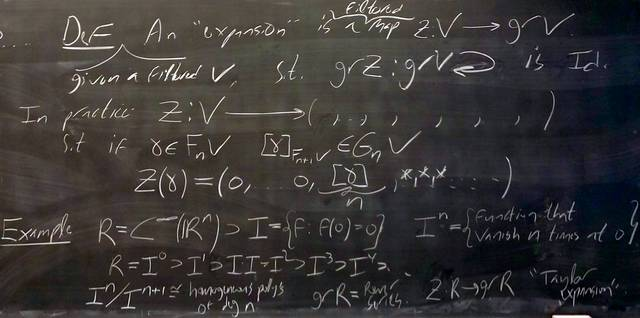170214-113531: Expansions, Taylor exapansions.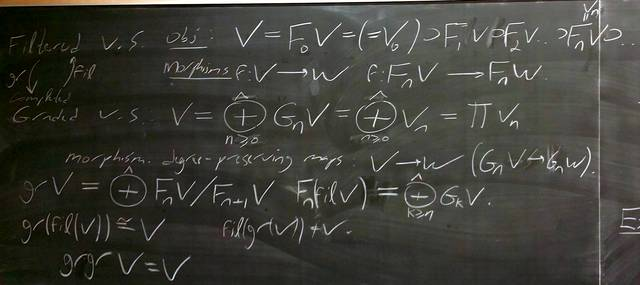170214-113525: The filtered category and the graded category.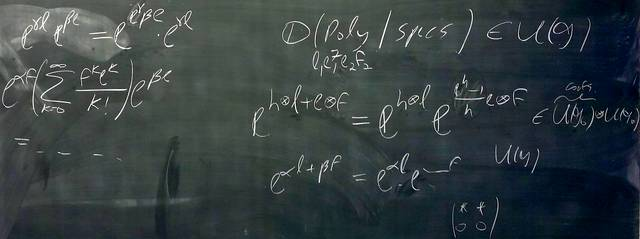170210-120611: Random notes for Feb 10.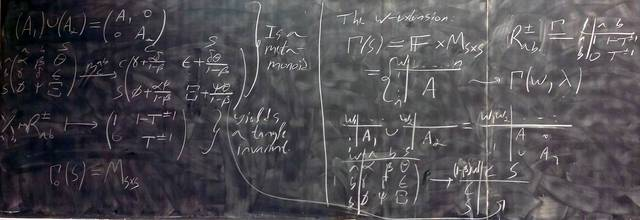170207-124944: $\Gamma_0$ and $\Gamma$ calculi.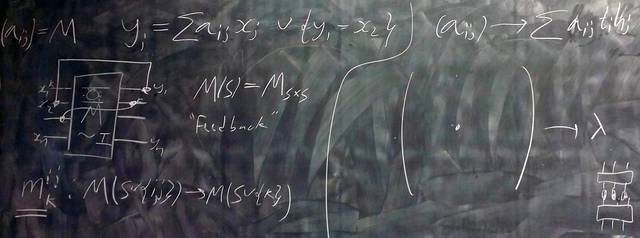170207-121605: The Linear Control Theory meta-monoid.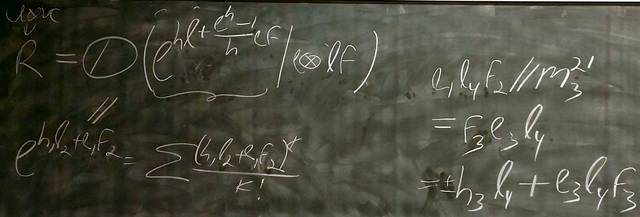170203-120318: The normal ordering operator ${\mathbb O}$.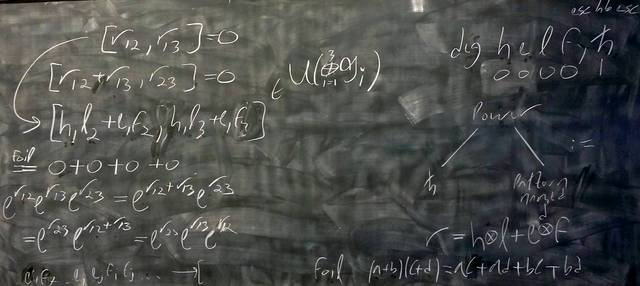170203-120305: A proof of YB for ${\mathfrak g}_0$.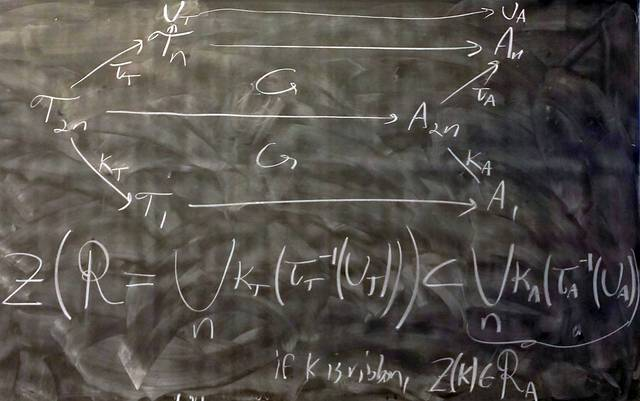170131-130125: A commutative diagrams for non-ribbon detection.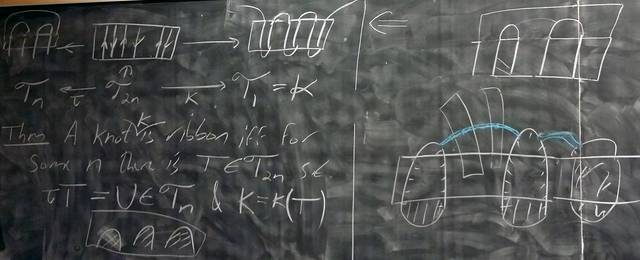170131-130119: Ribbons and tangles.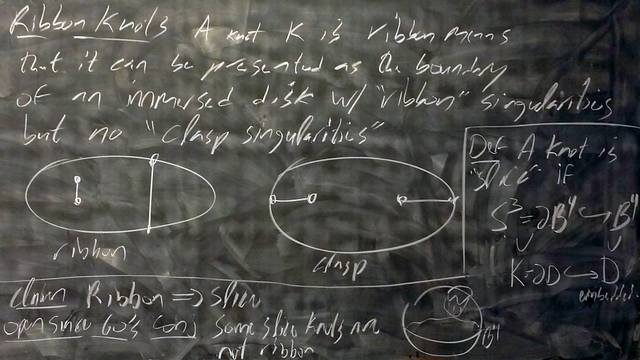170131-124305: Ribbon and slice.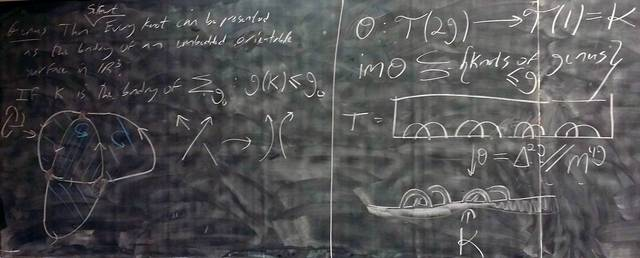170131-124300: Seifert surfaces and genus.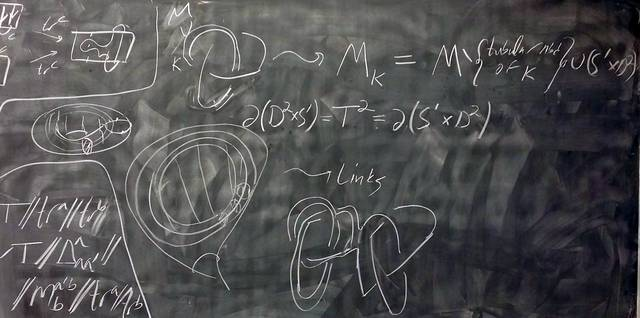170131-120335: Surgery and slides.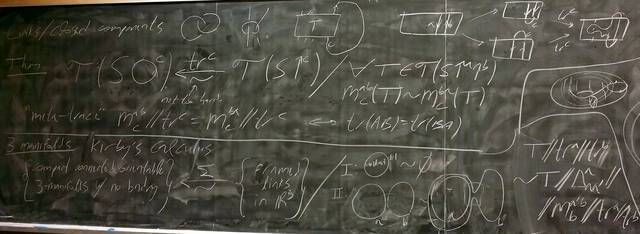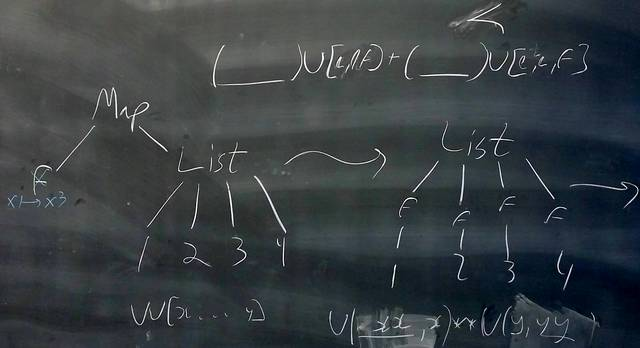170127-120518: Some Mathematica.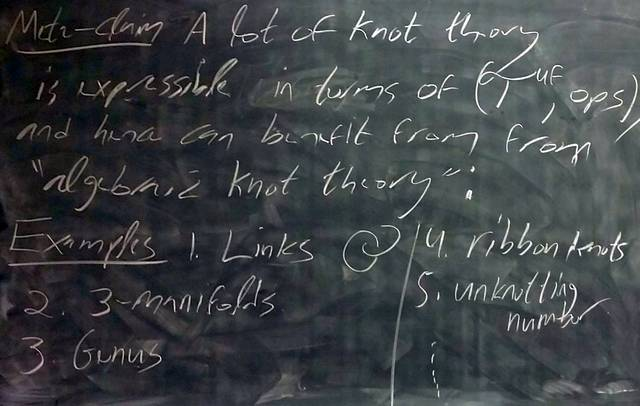170124-130009: The meta-claim of Algebraic Knot Theory.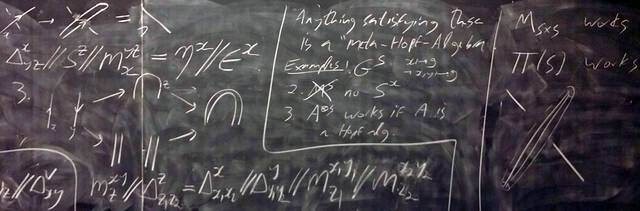170124-125255: Properties of these operations.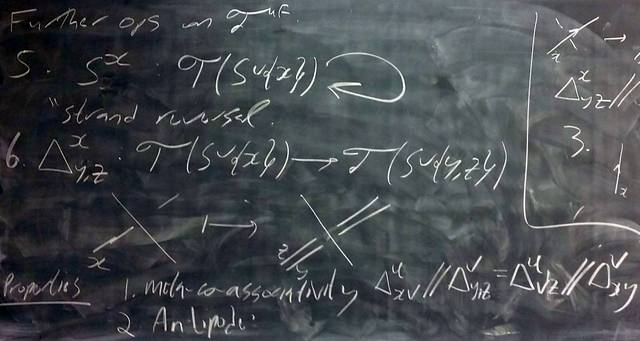170124-125249: Further operations on tangles.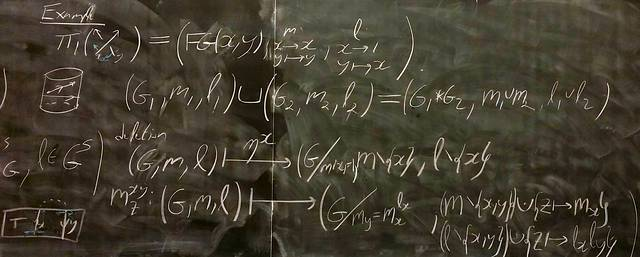170124-123253: The meta-monoid $\Pi$ (2).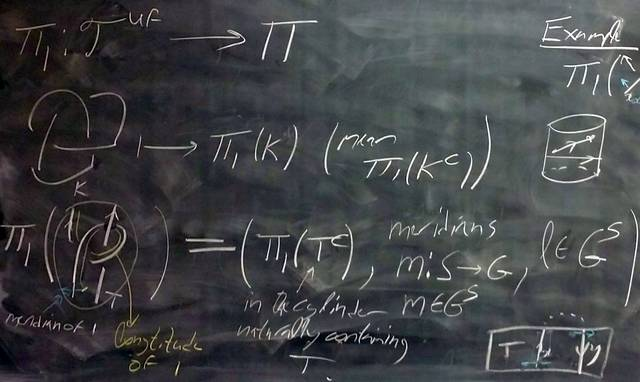170124-123246: The meta-monoid $\Pi$.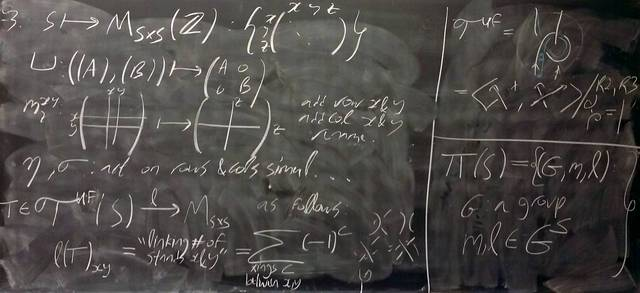170124-120130: Examples of meta-monoids.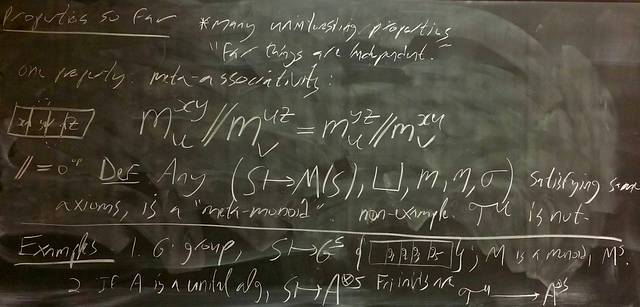170124-120121: Properties of these operations.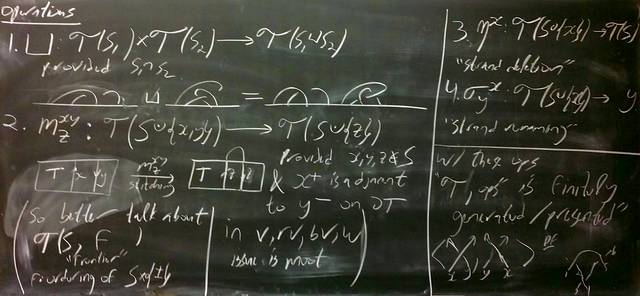170124-113422: Operations on tangles.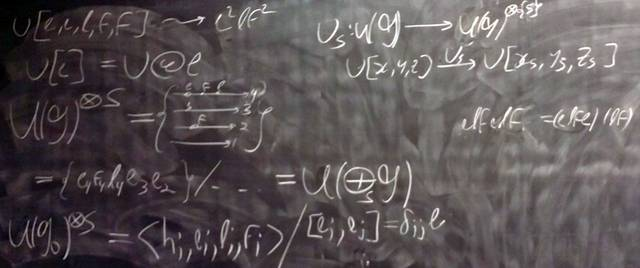170120-120257: Implementing PBW.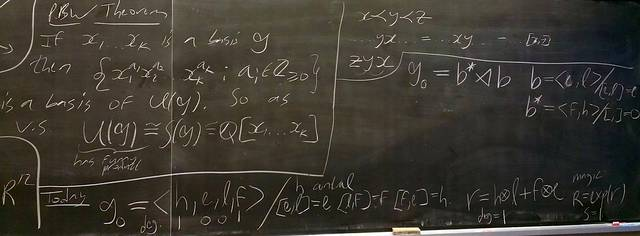170120-113723: PBW and ${\mathfrak g}_0$.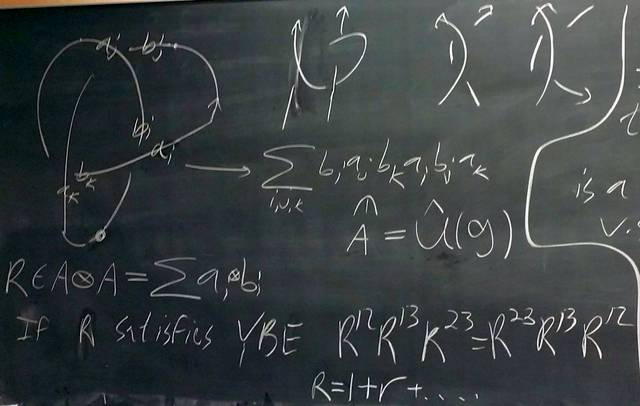170120-113715: The general scheme.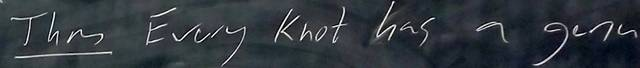170117-130246: The genus of a knot (2).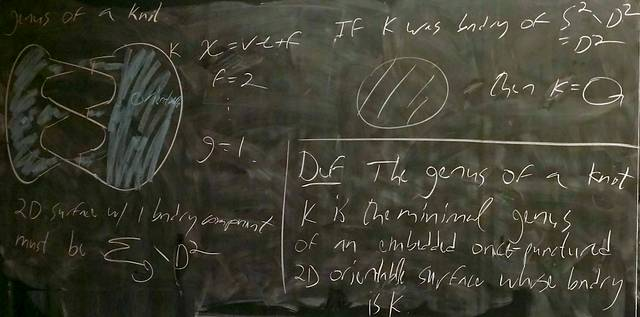170117-130245: The genus of a knot.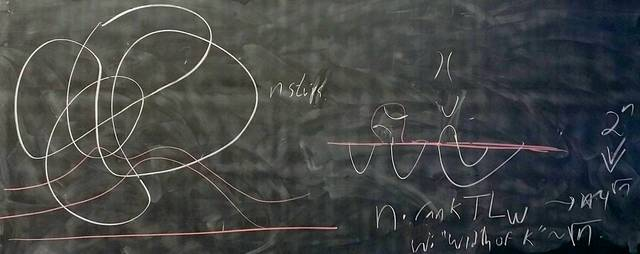170117-125113: The "Scan" algorithm.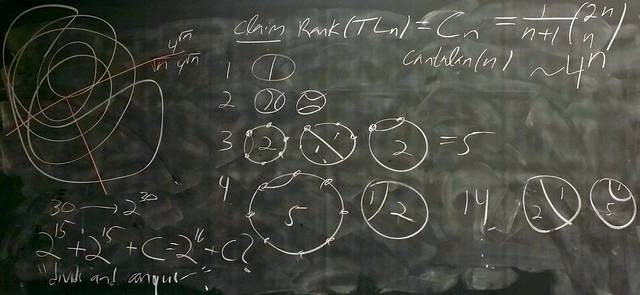170117-123835: Tangles and Temperley-Lieb (2).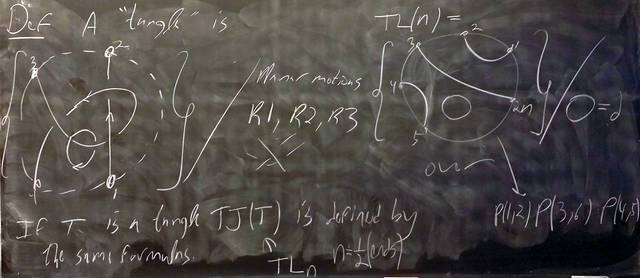170117-120423: Tangles and Temperley-Lieb.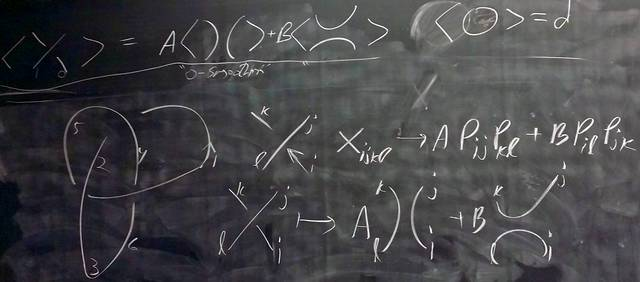170117-120414: Implementation note.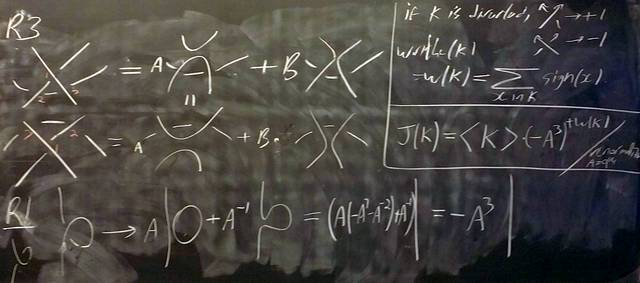170117-114030: Kauffman's presentation of the Kauffman bracket (2).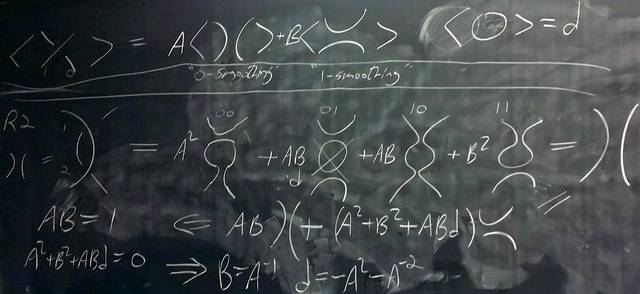170117-114025: Kauffman's presentation of the Kauffman bracket.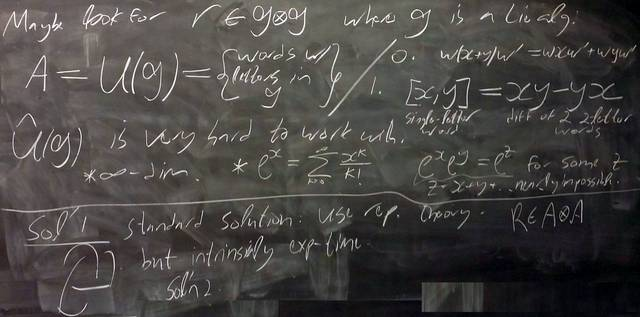170113-120253: Lie algebras and Universal Enveloping Algebras.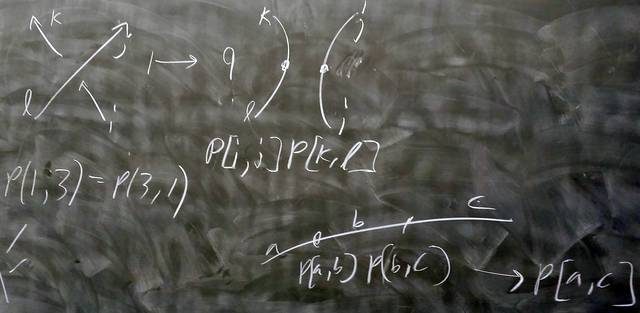170110-130144: The Jones program.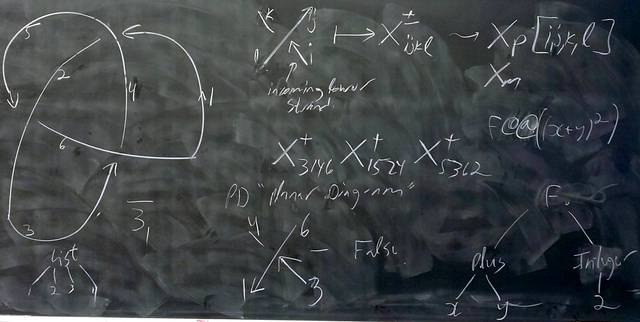170110-124647: Representing a knot on the computer, some Mathematica.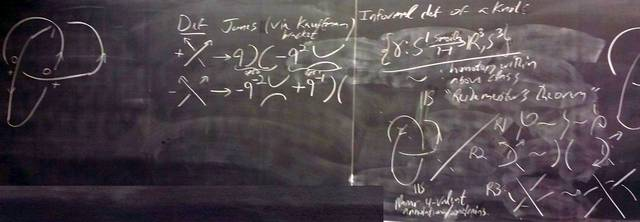170110-115829: Reidemeister moves and the Jones polynomial.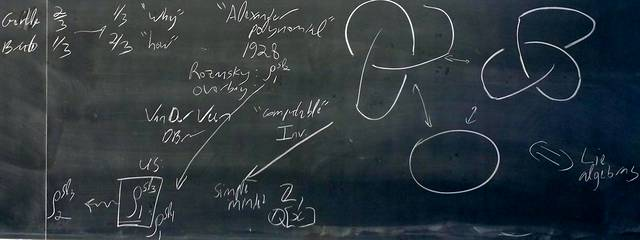170110-114004: What this class is about.